Courses

# Test: Complementary Angles of Trigonometry

## 15 Questions MCQ Test Mathematics (Maths) Class 10 | Test: Complementary Angles of Trigonometry

Description
This mock test of Test: Complementary Angles of Trigonometry for Class 10 helps you for every Class 10 entrance exam. This contains 15 Multiple Choice Questions for Class 10 Test: Complementary Angles of Trigonometry (mcq) to study with solutions a complete question bank. The solved questions answers in this Test: Complementary Angles of Trigonometry quiz give you a good mix of easy questions and tough questions. Class 10 students definitely take this Test: Complementary Angles of Trigonometry exercise for a better result in the exam. You can find other Test: Complementary Angles of Trigonometry extra questions, long questions & short questions for Class 10 on EduRev as well by searching above.
QUESTION: 1

### If tan 2A = cot (A – 18°), then the value of A is

Solution: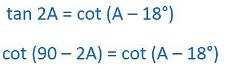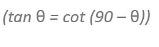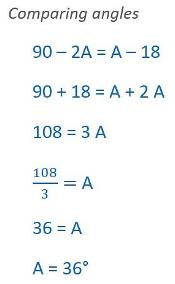QUESTION: 2

### The value of cos2 17° – sin2 73° is

Solution:

cos217-sin273
=cos217-sin2(90-17)
=cos217-cos217   (because sin(90-x)=cos x)
=0

QUESTION: 3

### If sec 4A = cosec (A-20°),where 4A is an acute angle, find the value of A

Solution: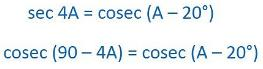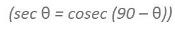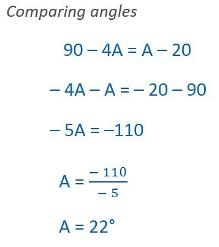QUESTION: 4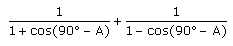​=

Solution: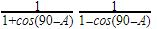Taking LCM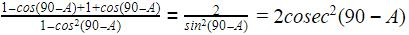QUESTION: 5

The value of cos θ cos(90° - θ) – sin θ sin (90° - θ) is:

Solution:
QUESTION: 6

Out of the following options, the two angles that are together classified as complementary angles are

Solution:
QUESTION: 7

The value of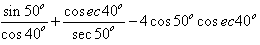is

Solution:

we know sin(90 - a) = cos(a)

cos(90 - a) = sin(a)

sin(a) = 1/cosec(a)

sec(a) = 1/cos(a)

cos40 = cos(90-50) = sin50

cosec40 = cosec(90-50) = sec50

so our expression becomes

sin50/sin50 + sec50/sec50 - 4cos50 / sin40

= 1 + 1 - 4(1)   since cos50 = sin40

= -2

QUESTION: 8

If cos (40° + A) = sin 30°, the value of A is:​

Solution:
QUESTION: 9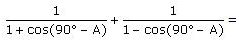Solution: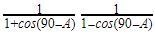Taking LCM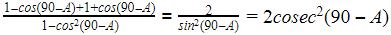QUESTION: 10

The value of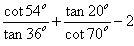is

Solution:
QUESTION: 11

sin (60° + θ) – cos (30° – θ) is equal to (where (60° + θ) and (30° - θ) are both acute angles):

Solution:
QUESTION: 12

The value of tan1°.tan2°.tan3°………. tan89° is :

Solution:

tan 1.tan 2.tan 3...tan (90 - 3 ).tan ( 90 - 2 ).tan ( 90 - 1)
=tan 1.tan 2 .tan 3...cot 3.cot 2.cot 1
=tan 1.cot 1.tan 2.cot 2.tan 3.cot 3 ... tan 89.cot 89
1 x 1 x 1 x 1 x ... x 1 =1

QUESTION: 13

Using the ratio of complementary angles, the value of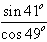is

Solution:
QUESTION: 14

If x sin (90° – θ) cot (90°- θ) = cos (90° – θ) then x is

Solution:
QUESTION: 15

If A and B are the angles of a right angled triangle ABC, right angled at C, then 1+cot2A =​

Solution:
ABC is a Δ, right angle at c.
1 +cot^2 =?........
we know that.....
Cosec^2 - cot^2= 1...
So,
=> 1+ cot^2
=> cosec^2 A
=> (AB)^2/( CB)^2
= sec ^2B.